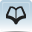# Resource 1: Number bond gamesTeacher resource for planning or adapting to use with pupils

Here are the instructions for the question and answer match game. Below are some examples of questions and answers. You could either copy these or ask your pupils to draw the squares themselves.

1. Cut up each square separately.
2. 2–6 players can play this game at any one time.
3. Place all the cards face down on the table. Keep the answers and questions separate to help the players.
4. Decide who goes first. Each player takes it in turn to turn over two cards – one from the sums first and then one from the answers. If the answer is right for the sum the player calls the first ‘match’. If they get a match, they can have another go. If not, the next player has their turn and does the same. Carry on in this way until all the sums are answered. The winner is the one who has most matches.
5. You could make this more challenging for older pupils by using more difficult sums, which include subtraction, multiplication or division. You will have to adapt the ‘answers’ accordingly.

## Examples of questions/sums

 1+0= 0+2= 1+1= 2+0= 1+2= 2+1= 0+4= 1+3= 3+1= 2+2= 0+5= 4+1= 1+4= 2+3= 3+2= 0+6= 1+5= 5+1= 2+4= 4+2= 3+3= 0+7= 1+6= 2+5= 5+2= 3+4= 4+3= 8+0= 1+7= 2+6= 6+2= 3+5= 4+4= 0+9= 8+1= 2+7= 3+6= 4+6= 7+3= 9+1=